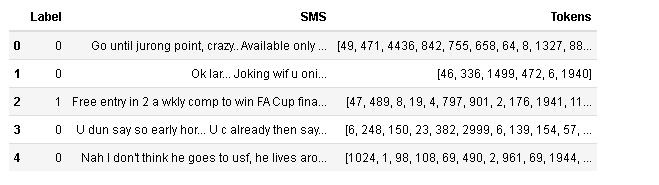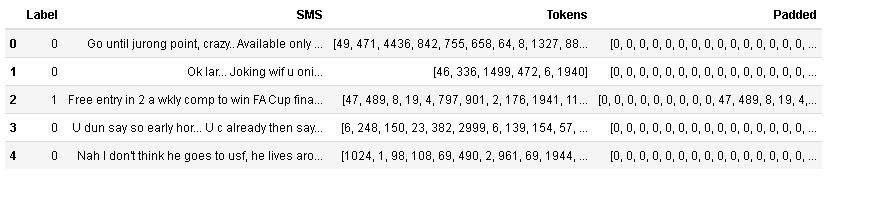# Deep Learning with Keras – Part 6: Textual Data Preprocessing

0
5634Image by Ahmed Gad from Pixabay

## Intro

Congratulations for going far in this Keras tutorial. After working with numeric, categorical and image data, it is time to handle textual data. Deep learning has proved itself in being very effective with Natural Language Processing (NLP) tasks. Before we dive into how to build NLP models, let us learn how to handle and preprocess textual data with Keras.

We already know that any machine learning model needs data to represented in a numeric format. Therefore, in this article we will learn how to load, preprocess, tokenize, convert and pad textual data into numeric sequences.

## Dataset

In the following coding exercises we will be playing with the Spam SMS dataset. The dataset is stored in a CSV file that contains two fields: Label and SMS. The SMS is the message text, while the label indicates whether the SMS is a spam or not. Kindly download from here, and load it as shown in the following code snippet:

``````import pandas as pd
data = pd.read_csv('../data/sms.csv')``````

Here is a look at the data:Initial dataset

## Tokenizing

Normally the first step in textual data preprocessing is splitting sentences into words/tokens. This could easily be done using the Keras tokenizer class.

``````from keras.preprocessing.text import Tokenizer
tokenizer = Tokenizer()
tokenizer.fit_on_texts(data['SMS'])
data['Tokens'] = tokenizer.texts_to_sequences(data['SMS'])``````

Let us check the results:Tokens

See how words got replaced by numbers! Well each word now has an index that represents it in a sentence.

In order to get what each index stands for, we can use the tokenizer index_word property:

``````print(tokenizer.index_word)
# output: go``````

We can go the other way around with the word_index property:

``````print(tokenizer.word_index['go'])
# output: 49``````

We have one more thing to take care of. See how each SMS has different number of words! This results in different sequence lengths; which may cause problems in some models. The solution is simple: Padding.

## Sequence Padding

Padding means adding zero values to the left or the right of a sequence. We simply decide the maximum sequence length and the position of the padding. But what if the sequence length is already greater than the maximum length? Well here just truncate! By the end we will have sequences with equal lengths. Here is how to do it:

``````from keras.preprocessing.sequence import pad_sequences
sequences = pad_sequences(data['Tokens'], maxlen=40, padding='pre', truncating='post')
data['Padded'] = sequences.tolist()``````

Simple right? Here are the final results:Padded sequences

## Conclusion

Well, you are all set! These are the basic tools for handling textual data in Keras. Next we will learn about a new deep learning architecture for NLP and sequential models in general. We will put these processed data into action while building our first efficient spam classifier. Stay tuned…

This site uses Akismet to reduce spam. Learn how your comment data is processed.